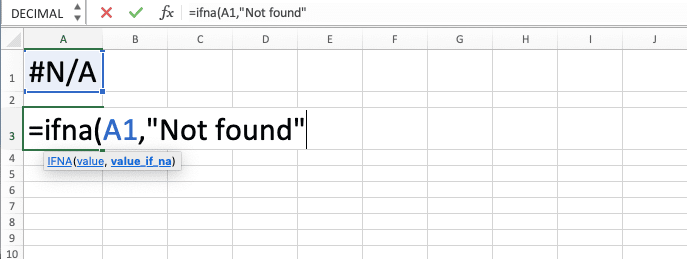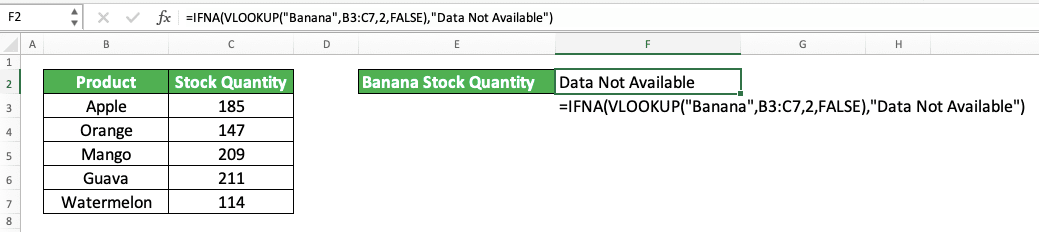How to Use Excel IFNA Function: Usabilities, Examples, and Writing Steps - Compute Expert

# How to Use Excel IFNA Function: Usabilities, Examples, and Writing Steps

Home >> Excel Tutorials from Compute Expert >> Excel Formulas List >> How to Use Excel IFNA Function: Usabilities, Examples, and Writing Steps

In this tutorial, you will learn about the excel IFNA function completely. We will discuss its usabilities, examples, writing steps, combination with another formula, and also its alternative.

When processing data in excel, we sometimes need to anticipate the errors we produce during our work. There are several types of errors in excel and IFNA can anticipate for us one error type that often shows up.

Disclaimer: This post may contain affiliate links from which we earn commission from qualifying purchases/actions at no additional cost for you. Learn more

## What is the IFNA Function in Excel?

IFNA is a function that can catch the #N/A error in excel and substitute the error value with another value.

#N/A is the error code for the “Not Available” error. This means excel cannot find the data we want in a specified cell range. Excel lookup formulas are usually the ones that produce this error as their main function is to find data for us.

## IFNA Usability

IFNA can help us to replace a #N/A error with another value if the error happens.

## IFNA Result

IFNA will produce our original value if it doesn’t find the #N/A error value in it. If it finds the error, however, then it will produce the substitute value that we input into it.

## Excel Version from Which We Can Use IFNA

We can use IFNA since excel 2013.

## The Way to Write It and Its Input

Here is the general writing form of IFNA in excel.

= IFNA ( something_to_anticipate_the_na_error_from , substitute_value )

We usually input a lookup formula into IFNA as we usually anticipate the #N/A error from it. However, you can input any kind of data or formula you want as your first IFNA input.

You can also input anything you want as the IFNA substitute value if the #N/A error happens. You should input something that can help to make your data processing still go smoothly despite the error occurrence.

## Example of Its Usage and Result: If NA Then 0

Here is an implementation and result example of IFNA in excel. Here, we try to substitute the #N/A error we find with 0.In the example, we use HLOOKUP to find the banana’s stock quantity from the table on the left. We get a #N/A error because there is no banana in the table products.

Using IFNA, we can change the #N/A error to 0. Just input the HLOOKUP result and the 0 in the IFNA and we will immediately get the #N/A substitution!

We can also do it in one writing if we want by placing the HLOOKUP directly as the IFNA input.

## Writing Steps

After discussing the IFNA writing form, inputs, and example, now let’s discuss its writing steps in excel. You can see the writing step implementation example in a screenshot so you can understand it easier.

1. Type an equal sign ( = ) in the cell where you want to put the IFNA result in2. Type IFNA (can be with large and small letters) and an open bracket sign after =3. Input the data or formula from which you anticipate the #N/A error. Then, type a comma sign ( , )4. Input the #N/A error substitution value. IFNA will produce this value if the data or formula you inputted previously has a #N/A error value5. Type a close bracket sign6. Press Enter
7. Done!## Using IFNA with Another Formula: IFNA VLOOKUP

As we mentioned in the IFNA example discussion, we can write IFNA and another formula in one formula writing. In fact, it is probably the most common practice if we use IFNA.

Regarding the formula we combine with IFNA, the most often one might be VLOOKUP. It is a popular lookup formula in excel and we sometimes need to anticipate the #N/A error it produces.

We usually write IFNA and VLOOKUP simultaneously by using this writing form.

= IFNA ( VLOOKUP ( lookup_reference , reference_cell_range , result_column_index , lookup_mode ) , na_substitute_value )

We write our VLOOKUP inside IFNA to anticipate the #N/A error it might produce. If it does produce the error, then IFNA will substitute it with the value we input.

Here is the IFNA and VLOOKUP combination implementation example in excel.We combine IFNA and VLOOKUP in the example to anticipate if VLOOKUP doesn’t find the stock quantity we want. Here, we try to find the banana stock quantity (again). But since it isn’t there on the left table, our VLOOKUP produces a #N/A error.

However, since we have already combined the VLOOKUP with IFNA, we produce another value besides #N/A instead. The IFNA substitute value here is a “Data Not Available” text and thus, this is what our IFNA VLOOKUP combination produces.

If the VLOOKUP does find the data, then we will get a normal VLOOKUP result instead of the substitute value.

## How to Catch All Types of Error, Not Just #N/A Error: IFERROR

IFNA can only anticipate the #N/A error for us. What if we want to anticipate not just #N/A, but all other types of error as well? For this, we can use the IFERROR formula.

The general writing form of IFERROR in excel is similar to IFNA.

= IFERROR ( something_to_anticipate_the_error_from , substitute_value )

Here is the implementation example of IFERROR in excel. We compare it with the IFNA implementation here.In the example, you can see how IFERROR can anticipate more error types than IFNA. Using IFNA for an error other than #N/A will still produce the error instead of the IFNA alternative value.

Thus, when you need to anticipate all types of errors, you should use IFERROR. However, if you need to know whether the error is #N/A or not, use IFNA to help you.

## Exercise

After you have learned how to use IFNA in excel, let’s deepen your understanding by doing the following exercise.

### Instructions

1. Copy all data in the NA 1 column to No. 1 column. Replace all the #N/A there with “Not Found”!
2. Add all data in the NA 2 column with 1000 in the No. 2 column. Assume all the #N/A there is 0!
3. Add all parallel data in the NA 3 and NA 2 columns and write the results in the No. 3 column. Assume all the #N/A in the NA 2 column is 0 and the NA3 column is 1000!

Besides HLOOKUP and VLOOKUP, the lookup formulas we usually use in excel are LOOKUP, INDEX MATCH, and XLOOKUP. You might also need IFNA when using one of these formulas to anticipate their #N/A error.

Related tutorials you should learn:

Get updated excel info from Compute Expert by registering your email. It's free!